Next: Worked Examples Up: Magnetic Induction Previous: The Alternating Current Motor

The Direct Current Motor

In steady-state, an AC motor always rotates at the alternation frequency of its power supply. Thus, an AC motor powered by the domestic mains supply rotates at 60Hz in the U.S. and Canada, and at 50Hz in Europe and Asia. Suppose, however, that we require a variable speed electric motor. We could always use an AC motor driven by a variable frequency AC power supply, but such power supplies are very expensive. A far cheaper alternative is to use a DC motor driven by a DC power supply. Let us investigate DC motors.

A DC motor consists of the same basic elements as a DC electric generator: i.e., a multi-turn coil which is free to rotate in a constant magnetic field. Furthermore, the rotating coil is connected to the external circuit in just the same manner as in a DC generator: i.e., via a split-ring commutator which reverses the polarity of the coil with respect to the external circuit whenever the coil passes through the plane perpendicular to the direction of the magnetic field. Suppose that an external DC voltage source (e.g., a battery, or a DC generator) of emfis connected across the motor. The voltage source drives a steady current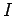around the external circuit, and through the motor. As the current flows around the coil, the magnetic field exerts a torque on the coil, which causes it to rotate. Let us suppose that the motor eventually attains a steady-state rotation frequency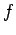. The rotating coil generates a back-emf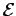whose magnitude is directly proportional to the frequency of rotation [see Eq. (222)].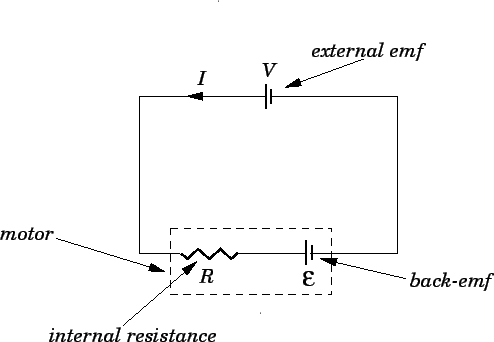Figure 44 shows the circuit in question. The motor is modeled as a resistor, which represents the internal resistance of the motor, in series with the back-emf. Of course, the back-emf acts in the opposite direction to the external emf. Application of Ohm's law around the circuit gives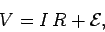(228)

which yields(229)

The rate at which the motor performs mechanical work is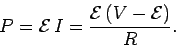(230)

Suppose that a DC motor is subject to a light external load, so that it only has to perform mechanical work at a relatively low rate. In this case, the motor will spin up until its back-emfis slightly less than the external emf, so that very little current flows through the motor [according to Eq. (229)], and, hence, the mechanical power output of the motor is relatively low [according to Eq. (230)]. If the load on the motor is increased then the motor will slow down, so that its back-emf is reduced, the current flowing through the motor is increased, and, hence, the mechanical power output of the motor is raised until it matches the new load. Note that the current flowing through a DC motor is generally limited by the back-emf, rather than the internal resistance of the motor. In fact, conventional DC motors are designed on the assumption that the back-emf will always limit the current flowing through the motor to a relatively small value. If the motor jams, so that the coil stops rotating and the back-emf falls to zero, then the currentwhich flows through the motor is generally so large that it will burn out the motor if allowed to flow for any appreciable length of time. For this reason, the power to an electric motor should always be shut off immediately if the motor jams. When a DC motor is started up, the coil does not initially spin fast enough to generate a substantial back-emf. Thus, there is a short time period, just after the motor is switched on, in which the motor draws a relatively large current from its power supply. This explains why the lights in a house sometimes dim transiently when a large motor, such as an air conditioner motor, is switched on.

Suppose that a DC motor is subject to a constant, but relatively light, load. As mentioned above, the motor will spin up until its back emf almost matches the external emf. If the external emf is increased then the motor will spin up further, until its back-emf matches the new external emf. Likewise, if the external emf is decreased then the motor will spin down. It can be seen that the rotation rate of a DC motor is controlled by the emf of the DC power supply to which the motor is attached. The higher the emf, the higher the rate of rotation. Thus, it is relatively easy to vary the speed of a DC motor, unlike an AC motor, which is essentially a fixed speed motor.Next: Worked Examples Up: Magnetic Induction Previous: The Alternating Current Motor
Richard Fitzpatrick 2007-07-14